Pure and Applied Mathematics Journal
Volume 4, Issue 5-1, October 2015, Pages: 28-32

An Approach for Intrusion Detection of IPv6 Network Based on LS-SVM Algorithm

Liu Jing1, 2

1College of Mathematics and Information Science, Weinan Normal University, Weinan, P. R. China

2Research Center of Weinan Wisdom City Engineering Technology, Weinan Normal University, Weinan, P. R. China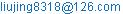(Liu Jing)

Liu Jing. An Approach for Intrusion Detection of IPv6 Network Based on LS-SVM Algorithm. Pure and Applied Mathematics Journal. Special Issue: Mathematical Aspects of Engineering Disciplines. Vol. 4, No. 5-1, 2015, pp. 28-33. doi: 10.11648/j.pamj.s.2015040501.16

Abstract: IPv6 has enough IP addresses to solve the problem of lack of IP address space. However, there are many security problems to be concerned. The detection ability of current intrusion detection system is poor when given less priori knowledge. In this paper, we analyze the Least Squares Support Vector Machine (LS-SVM) algorithm and the working process of snort intrusion detection system. And then we study the methods of intrusion detection in IPv6, and use LS-SVM to optimize snort intrusion detection system. Simulation results show that intrusion detection system with LS-SVM has a robust performance and has high detection efficiency.

Keywords: Intrusion Detection, Least Squares Support Vector Machine, IPv6, Snort

1. Introduction

Currently, as an effective solution to network IP address space exhaustion issue, IPv6 has become more popular. Security of IPv6 has also improved over IPv4 protocol network, but there are many security problems to be concerned (see [1,2]).

As a proactive network security defenses, intrusion detection can not only implement the internal attacks and external attacks by monitoring the network, but also effectively compensate for the lack of the firewall. However, precision of intrusion detection system is low in the case of lack of prior knowledge (see ). We get a lot of IPv6 protocol network operation logs and records by using the sort intrusion detection system (see ), and think of process of snort intrusion detection system as multi-class classification. Then we proposed that using Least Squares Support Vector Machine (LS-SVM) to optimize snort intrusion detection system. In this way, the data after preprocessing are classified, and intrusion detection system using a smaller sample ensures better detection performance with less of prior knowledge.

We now outline the remainder of this paper. In Section 2, a brief overview of the Least Squares Support Vector Machine was given. In Section 3, a snort intrusion detection system using LS-SVM was modeled. In Section 4, a simulation was designed and the results were analyzed.

2. Least Squares Support Vector Machine

Least Squares Support Vector Machine (LS-SVM) is an evolutionary algorithm based on Support Vector Machine(SVM). It uses the method of LS-SVM instead of the standard SVM quadratic programming method, converts quadratic optimization problem with inequality constraints original space into the equation kernel space constraints, and converts the standard SVM inequality constraints into equality constraints by solving linear equations to obtain the least squares support vector machine classifier model. In this way, the solution process is transformed to solve linear equations. Since the complexity of algorithm is low and the efficiency is high, so we can apply LS-SVM to solve classification problems (see [5,6,7]).

2.1. The Model of LS-SVM

Suykens and Vandewalle proposed LS-SVM to solve the problem of classification and function estimation. The process of the model of LS-SVM is as follows (see [8,9]).

We assume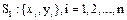as the training samples, where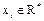is the classified sample vector,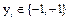is the corresponding number in the classified sample category,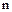is the capacity of classified samples. The inequality equation in the optimization constraints of SVM is replaced by equality equation, and empirical hazard function is converted into a quadratic function. Then we get the formula optimization problems shown in the Formula 2.1.(2.1)

In the Formula 2.1, the symbol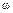is the direction vector in hyperplane,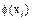is a mapping function from input sample space to feature space,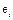is the relaxation factor of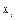, and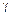is the marginal factor.

The solution of Formula 2.1 is shown in the Lagrange function in Formula 2.2.(2.2)

The symbol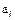is the Lagrange multiplier. We can get some results as follows by calculating Formula 2.2.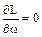,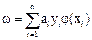,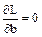,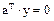,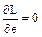,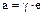,,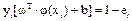and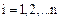.

We combine above formulas and solved the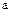and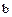by linear equations shown in Formula 2.3.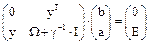(2.3)

The symbol I is an n-dimensional identity matrix in the Formula 2.3, E is an n-dimensional unit column vector,is the penalty factor used to balance the complexity of machine learning and experience risk, and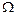is an n-dimensional symmetric square matrix. In addition,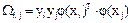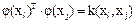,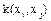is the kernel function. Therefore,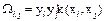is the i-th row j-th column element. Typical kernel functions are radial basis function and polynomial kernel function.

If we assume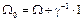, then the Formula 2.3 will be transformed as follows.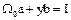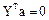After obtainedandby solving the transformed equation, we can obtain the LS-SVM classification function as shown in Formula 2.4.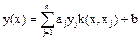(2.4)

We only need to calculate each kernel functionbetween training samples and test samples to get the model of LS-SVM classification without the specific form of the function. Verdict pattern vector x categories are decided by the discriminant function in Formula 2.5.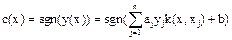(2.5)

Symbol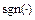in the Formula 2.5 is the sign function. When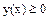,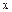is judged as positive category, otherwiseis judged as negative category.

2.2. Least Squares Support Vector Machine (SVM) Algorithm

By analyzing the mathematical principle of Least Squares Support Vector Machine (LS-SVM), the key to realize the classification model is to solve theandvalue, using Lagrange function, the problem can be transformed into solving linear system of equations, algorithm steps are as follows:

Algorithm 1: Least Squares Support Vector Machine (LS-SVM) algorithm

(1) Input the training sample: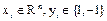,is the training sample,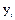is the classification of training data.

(2) Calculate the kernel function model. The commonly used kernel function is the same as the kernel function of SVM model selected.

(3) Calculate the kernel matrix. The calculation of the kernel matrix can be obtained by training data matrix, kernel function and kernel parameter. For each of the data matrix as input, set up the kernel values by the kernel function satisfied the Mercer condition, so the kernel matrix must be a positive definite matrix.

(4) Data normalization, calculate the α and b value. Input the training data matrix, calculate the coefficient matrix, solve the linear system of equations Formula 2.3, get the coefficient vector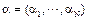and. The diagonal elements of the matrix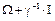is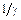, it can be verified that the matrixis a positive definite matrix, so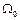=, the value ofandcan be calculated,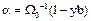,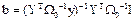. At first, calculate the value of, then can get the value of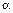,through these calculations, all the training samples have become support vector, this is the difference between the Least Squares Support Vector Machine (LS-SVM) and Support Vector Machine (SVM).

(5) Calculate the classification hyperplane and discriminant function. After get the α and b values, calculate the classification hyperplane as shown in Formula 2.4, the classification of X can be determined by the discriminant function of the trained classification hyper plane as shown in Formula 2.5.

Through the mathematical principle and algorithm steps of Least Squares Support Vector Machine (LS-SVM), solving a set of linear equations and obtain the optimal classification plane, coefficient matrix of linear equations is, the computational complexity is greatly simplified, the demand for storage space is greatly reduced, the computational efficiency is improved and the computational cost is greatly reduced.

2.3. LS-SVM Multi-classification Method

In order to make the LS-SVM can solve the classification problem of multi-class samples, here is a "one-to-one" algorithm combined with LS-SVM, then use the voting algorithm, the classification of the most votes is determined to be the class of sample points. The design process analysis is as follows:

To solve the classification problem of K class samples, the K class samples classification problem can be considered as a series of two types of classification problem. Use the "one-to-one" algorithm combined with LS-SVM method, and multi-classification LS-SVM can be built. For the K class classification problem, two classes of LS-SVM classifier is constructed for any two different classes. Each classifier is trained only on the two classes of training samples in the K class samples,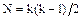classifiers need to be constructed.

In constructing of the two classes of LS-SVM classifier using class i and class j, respectively select the sample data belong to the class i and class j as the training samples. Samples belong to class i are labeled as positive, samples belong to class j are labeled as negative. The MaxWin algorithm is analyzed (see ). For each "one-to-one" LS-SVM classifier, assign it to the most likely class based on the Voting mechanism, the final result is the one with the largest number of votes, and it can be proved that the algorithm is Bayesian optimal.

Therefore, respectively use the number of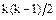two classes of LS-SVM classifiers to classify the test sample x, if x belongs to class i, then add one note to class i, otherwise add one note to class j, until all the classification is finished, the classification of the most votes is determined to be the class of test samples. The "one-to-one" algorithm based on LS-SVM is easy to implement and the training speed is fast.

3. Network Intrusion Detection of IPv6

IPv6 is a new IP protocol designed by IETF to replace IPv4, it was improved in address space, the data structure of header, address auto configuration, IPSec protocol and the quality of service. Header data structure and IPSec have main influence to the design and implementation of snort intrusion detection system, bring new changes to data analysis and detection (see ).

3.1. Snort Intrusion Detection System (Open Source)

Snort is a C language intrusion detection system run on the Libpcap library functions, consists of the following several modules, packet sniffer, preprocessor, detection engine and alarm output. Use rules matching mechanism to detect whether the network behavior in violation of the security policy configured in advance. The host installed Snort can monitor the shared segment. Once found the intrusion and detection behavior, it will take several alarm way to send the alarm message to system log, alarm files or console screen, etc., at the same time, it can record the intrusion behavior of host and network, and used for further analysis research. The process of snort including packet decoding, pretreatment, intrusion detection, alarm and logs, output, etc. After installation configuration and start the snort, the initialization completed. Snort explain the commands, read the rules database of the system, generate rules of two-dimensional chain table used in intrusion detection, and then fall into a process to circular caught and rule matching, once detected abnormal behavior, it will take warning or record actions of invasion. This paper is to analysis the alarm generated test data again, judge invasion behavior and discover new snort rules (see ).

3.2. The Characteristics of IPv6 Supported Network

3.3. Performance Evaluation Indicators of Intrusion Detection

The commonly used invasion detection performance evaluation mainly includes the detection rate, false alarm rate, accuracy, complexity, processing performance, completeness, fault tolerance and real-time performance, etc. In this paper, detection rate, accuracy and real-time performance were chosen as the evaluation standard. Firstly, measure the performance of the LS-SVM through detection rate. Detection rate is defined as the ratio of the number of detected attack in the invasion samples and all the invasion samples, this ratio is associated with false alarm rate, at the same detection rate, intrusion detection algorithm with low false alarm rate has better detection accuracy, or at the same false alarm rate, intrusion detection algorithm with high detection rate has better detection accuracy. Detection rate reflects recognition ability of intrusion detection model for attack, if the test sample contains new attacks, it reflects the recognition ability of intrusion detection algorithm for unknown attacks, and this is the scalability and adaptability of the intrusion detection algorithm. The higher is the detection rate, the better is the performance of intrusion detection algorithm. Secondly, analyzed the time complexity of the LS-SVM, investigate the time for data classification processing. In fact, we all hope the intrusion detection algorithm can response timely, in order to reduce the loss brought by the invasion. Thirdly, determine the accuracy of intrusion activities that the LS-SVM can detect, when an intrusion detection system is not accurate, it may mistake normal behavior for intrusion, then leads to false alarm.

4. Simulation Experiment and Result Analysis

The experiment based on snort2.9.4 intrusion detection system and Matlab7.1, use LS-SVMlab1.5 toolbox (see ), detect and recognize the KDD99 intrusion detection data set and intrusion detection data set using snort intrusion detection software.

4.1. Data Source

This experimental data comes from two aspects: 1.Host log data and network data acquired by snort2.9.4 intrusion detection.2.The Kdd data set.

Use snort2.9.4 to get the data set. Snort can record possible invasion information by using the text, XML, Libpcap format, also can output the message to the syslog or database. Brian Caswell wrote the CSV output plug-in for snort, so snort can record data by using CSV format, the format is as follows:

05/10-10:02:31.953089, INFO - ICQ Access, TCP, 10.1.1.1, 10.2.2.5.

This experiment output the data captured by the snort intrusion detection to the CAS file, converted to the standard format which Least Squares Support Vector Machine (LS-SVM) can recognized, then make the standard format as the training samples to do some classification and recognition.

Use the KDD99 data set. KDD data set (see ) originated in the U.S. Defense Advanced Research Projects Agency in 1998 and intrusion detection evaluation project carried out by Air Force Research Labs, consists of 7 week training data and 2 week test data, data are all taken from the real network data, by using the network data traffic, the host audit records and system files for dump. The experimental data is widely used in intrusion detection simulation; there are 5 million training data and 300000 test data.

About 3000000 of these attacks were extracted from 38 different attack types and 7 different attack scenarios, almost includes all kinds of normal or abnormal data, also contains the following four kinds of attack data, denial of service attack, unauthorized access to a remote machine, all kinds of authority promotion, all kinds of port scanning and vulnerability scanning, altogether contains 41 characteristics, including 32 data continuity characteristics, 9 discrete eigenvalues.

The format is as follows:

Normal data: 0,tcp, http,SF,159,4087,0,0,0,0,0,l,0,0,0,0,0,0,0,0,0,0,5,5,0.00,0.00,0.00, 0.00,1.00,0.00,0.00,11,79,1.00,0.00,0.09,0.04,0.00,0.00,0.00,0.00,normal.

Attack data: 0,icmp,ecr_i,SF,1032,0,0,0,0,0,0,0,0,0,0,0,0,0,0,0,0,0,511,511,0.00,0.00, 0.00,0.00,1.00,0.00,0.00,255,69,0.27,0.02,0.27,0.00,0.02,0.00,0.00,0.00,smurf.

4.2. Data Pre-processing

In order to use the LS-SVM model for data classification, the data set must be preprocessed. Convert the character data set into a continuous integer; convert the gained character net data set, such as protocol type, attack type into numerical values. The 41 character attributes used in the following simulation experiments were all converted into consecutive integers in the preprocessing, maintain the value of the data set, and then also ensure the data set is classified. We normalized the numerical ranges into interval [0,1]. This is because, when use the Least Squares Support Vector Machine (SVM) to calculate the distance numerical ranges, different numerical domain may cause different influence to distance, normalization can improve the classification accuracy. After the preprocessing, the data format is unified as the row vector of pure data, and set to 41 dimension numerical vector, using Xtrain, Ytrain, Xtest and Ytest to express.

4.3. Experiment and Analysis

In this experiment, the CPU parameters of host are Intel(R) Core(TM)2 Duo CPU T8100 2.10GHz, the memory parameter is DDR 1GB, the network card parameter is Intel(R) 82566MM Gigabit Network Connection, the OS is Windows XP SP3 32-bit, the intrusion detection system is snort2.9.4, the multi class algorithm development platform based on LS-SVM are Matlab7.1 and LS-SVMlab1.5 tool box.

Respectively set training function type parameters, kernel function type parameters, coding scheme, whether to preprocess the data, the value of gam and the value of sig to type='classifier'; kernel_type='RBF_kernel'; gam=1; sig2=10; preprocess='preprocess'; codefct='code_MOC';

Parts of the key code are as follows:

Yc, codebook, old_codebook] = code (Ytrain, codefct)

[alpha, b] = trainLS-SVM ({X, Yc, type, gam, sig2, kernel_type, preprocess})

Ydtemp = simLS-SVM ({X, Yc, type, gam, sig2, kernel_type, preprocess}, {alpha,b}, Xtrain)

Yhc = simLS-SVM ({X, Yc, 'classifier', gam, sig2, 'RBF_kernel', 'preprocess'}, {alpha,b}, Xtest)

Yd = code (Ydtemp, old_codebook, [], codebook)

SimResult =~abs (Yd-Ytest)

ClassifyPercent =sum (SimResult)/ length (SimResult)

6 groups experiment were carried out on two data sets, in each experiment, select 410 training vectors and 4100 test vectors randomly, the result is shown in tab. 1

Tab. 1. Intrusion detection results based on the LS – SVM.

 Experimental group The gained data set by Snort2.9.4 KDD intrusion detection data set Recognition rate (%) Time complexity (s) Recognition rate (%) Time complexity (s) 1 100.0 3.1 91.20 4.3 2 78.89 3.6 91.89 3.9 3 79.01 3.9 92.00 3.4 4 71.12 3.3 91.00 4.0 5 68.89 3.3 91.33 4.1 6 78.09 3.7 92.50 4.4

5. Conclusion

In this paper, we use snort intrusion detection system to analyze the security of IPv6. Based on the analysis of the LS-SVM algorithm and the working process of snort intrusion detection system, we use LS-SVM to optimize snort intrusion detection system. Simulation results show that intrusion detection system with LS-SVM can gain high detection performance.

Acknowledgement

This work was supported by the Young National Natural Science Fund Project under Grant No.61402335, The National Bureau of Statistics Research Projects under Grant No.2012LY056, Weinan Normal University Research Projects under Grant No.14YKS007, and Weinan Normal University Characteristic Discipline Construction Projects under Grant No.14TSXK02.

References

1. Qing SH,"research on intrusion detection techniques: a survey",JOURNAL OF CHINA INSTITUTE OF COMMUNICATIONS, 25(2004),19-29.
2. KUMAR S,Classification and Detection of Computer Intrusions,Dissertation, Purdue University, 1995.
3. Gomathy, A., and B. Lakshmipathi."Network intrusion detection using Genetic algorithm and Neural Network"Advances in Computing and Information Technology,Springer Berlin Heidelberg,(2011),399-408.
4. The open source network intrusion detection system [EB/OL],http://www.snort.org/.
5. Suykens J A K, Vandewalle J, "Least Squares Support Vector Machine Classifiers",Neural Processing Letters,9(3)(1999),293-300.
6. P.H. Chen, R.E. Fan, and C.J. Lin,A study on SMO-type decomposition methods or support vector machines. IEEE Transactions on Neural Networks,(2006).
7. J.A.K.Suykens, T.Van Gestel, J.De Brabanter, B.De Moor, J.Vandewalle, "Least Squares Support Vector Machines".Singapore: World Scientific publishing,(2002).
8. Suykens J A K, Vandewalle, De Moor B,Optimal Control by Least Squares Support Vector Machines. Neural Networks,14(1)(2001),23-35.
9. Wang, Haifeng, and Dejin Hu,"Comparison of SVM and LS-SVM for regression",Neural Networks and Brain(2005).
10. Friedman J H.,"Another Approach to Polychotomous Classification",Technical Report. Standford University. Depart-ment of Statistics,10(1998),1895-1924.
11. Deering S,Hinden R,Internet Protocol Version 6 (IPv6) Specification,IETF,12(1995).
12. Andrew R. Baker,Joel Esler, "Snort Intrusion Detection and Prevention Toolkit",Syngress Publishing, Inc.,(2007).
13. Martin Roesch,Chris Green,Sourcefire. SNORT Users Manual 2.9.4,11(2012).
14. Erana, E. I. and Scheffer, T."IPv6 Intrusion De-tection mit Snort",In Forschungsbericht der Beuth Hochschule fur Technik Berlin,Beuth Verlag GmbH Berlin-Wien-Zurich (2010).
15. Hogg, S. and Vyncke, E.,IPv6 Security. Cisco Press,Indianapolis, IN 46240 USA.,(2009).
16. Loshin, Pete."IPv6: Theory, Protocol, and Practice". San Fransisco: Morgan Kaufmann Publishers,(2003).
17. Kent S.IP encapsulating security payload (ESP), RFC4203 [EB/OL]. http://www.ietf. org/rfc/rfc4203.txt,(2005).
18. K. Pelckmans, J.A.K. Suykens, T. Van Gestel,et.al,Vandewalle,LS-SVMlab Toolbox User’s Guide.http://www.esat.kuleuven.ac.be/sista/LS-SVMlab.
19. KDD Cup 99 DATA.http://kdd.ics.uci.edu.

 Contents 1. 2. 2.1. 2.2. 2.3. 3. 3.1. 3.2. 3.3. 4. 4.1. 4.2. 4.3. 5.
Article ToolsAbstractPDF(207K)